Zum Inhalt springen
• de
• en
Menü

Wir verwenden Google für unsere Suche. Mit Klick auf „Suche aktivieren“ aktivieren Sie das Suchfeld und akzeptieren die Nutzungsbedingungen.

###### FSofSG(TR,alpha)- Features surfaces of SG% FSofSG(TR,alpha) - Features surfaces of SG% (by Tim Lueth, VLFL-Lib, 2017-JUL-09 as class: SURFACES)% % This fnctn can take several seconds to classify all OPEN surfaces of a % solid by using TR3neighborsAngle and neighborsAngleSurface.% When called without output parameter; drawing the surfaces take the % most time.% SGsurfaces returns CLOSED surfaces (Status of: 2017-07-09)% % Introduced first in SolidGeometry 4.0% % See also: surfacesofSG% % FIL=FSofSG(TR,[alpha])% === INPUT PARAMETERS ===% TR: Solid Geometry or Triangulation% alpha: maximum angle difference between two facets; default is 1 ~ 60 % degree% === OUTPUT RESULTS ======% FIL: Index List for the Surface Indices% % EXAMPLE: % load JACO_robot.mat% surfacesofSG(JC3);% % See also: surfacesofSG%
###### VLmeshgrid(xx,yy,zz)- simple function for a better understand of meshgrid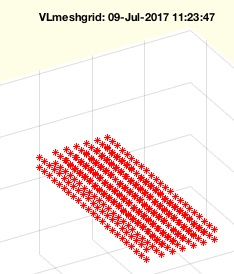% VLmeshgrid(xx,yy,zz) - simple fnctn for a better understand of meshgrid% (by Tim Lueth, VLFL-Lib, 2017-JUL-09 as class: AUXILIARY PROCEDURES)% % Introduced first in SolidGeometry 4.0% % See also: CPLofmeshgrid(X, Y), PLmeshgrid% % VL=VLmeshgrid(xx,yy,[zz])% === INPUT PARAMETERS ===% xx: list of x values% yy: list of y values% zz: list of y values; % === OUTPUT RESULTS ======% VL: Point list of all mesh coordinates% % EXAMPLE: % VLmeshgrid(1:3,1:2,10)% VLmeshgrid([0 15 25 35 45 55 65],0:8:8*22,[10 20])% % See also: CPLofmeshgrid(X, Y), PLmeshgrid%
###### VLedgeNormal(VL,ez)- returns the normal vector to vertex edge list% VLedgeNormal(VL,ez) - returns the normal vector to vertex edge list% (by Tim Lueth, VLFL-Lib, 2017-JUL-09 as class: EDGE LISTS)% % It does not support nan separted vertex lists (CVL) yet. The normal is % NaN for the first point or the given ez. The normal is NaN for the % lastpoint. The normal is NaN for all straight points. In case of % switching normals; the orientation of the succeeding normal will be % fliped. (Status of: 2017-07-09)% % Introduced first in SolidGeometry 4.0% % See also: VLFLfaceNormal, edgeNormal, CPLedgeNormal% % [NL,AL]=VLedgeNormal(VL,[ez])% === INPUT PARAMETERS ===% VL: Vertex list of succeeding vertices% ez: optional first normal vector; default is normal of facet [1 2 3]% === OUTPUT RESULTS ======% NL: Normal vector; NaN if A(i)=0;% AL: area vector; if straight, A(i)=0;% % EXAMPLE: % VL=VLsample(12); VL=[VL;VL(1:2,:)]; VLedgeNormal(VL)% VLedgeNormal(PLhelix(10,10,4*pi))% VLedgeNormal(VLsample(9))% % % See also: VLFLfaceNormal, edgeNormal, CPLedgeNormal%
###### PLmeshgrid(xx,yy)- simple function for a better understand of meshgrid% PLmeshgrid(xx,yy) - simple fnctn for a better understand of meshgrid% (by Tim Lueth, VLFL-Lib, 2017-JUL-08 as class: AUXILIARY PROCEDURES)% % Introduced first in SolidGeometry 4.0% % See also: VLmeshgrid, CPLofmeshgrid(X, Y)% % PL=PLmeshgrid(xx,yy)% === INPUT PARAMETERS ===% xx: list of x values% yy: list of y values% === OUTPUT RESULTS ======% PL: Point list of all mesh coordinates% % EXAMPLE: % PLmeshgrid(1:3,1:2)% PLmeshgrid([0 15 25 35 45 55 65],0:8:8*22)% % See also: VLmeshgrid, CPLofmeshgrid(X, Y)%
###### SGgrowFS (SG,t)- grows the feature surfaces of a solid% SGgrowFS (SG,t) - grows the feature surfaces of a solid% (by Tim Lueth, VLFL-Lib, 2017-JUL-08 as class: SURFACES)% % The feature surfaces are calculated (default value is 1 rad/60 degree). % Afterwards it is analyzed wich facets show inside of the solid an which % show outside. Only inside or outside are processed here. Use SGgrow for % processing all. At the border between outside and inside, the vertices % that belong to both (inside, outside) stay unchanged. The vertices that % define the border of the new outside surfaces are not moved along the % vertex normal vector but along the normal vector of the border contour!% Attention: Be aware that concave surface cannot be grown without % self-penetration. (Status of: 2017-07-08)% % Introduced first in SolidGeometry 4.0% % See also: SGgrowFS, SGgrow% % SGgrowFS(SG,[t])% === INPUT PARAMETERS ===% SG: Solid Geometry% t: Increase/Decrease Value along the vertex normal vector% % EXAMPLE: % load JACO_robot.mat % use loadweb if the file need to be aquired% SG=SGseparate(JC3,3)% SGgrowFS(SG,10)% % See also: SGgrowFS, SGgrow%
###### clipboardrem(maxc)- removes comment characters from the clipboard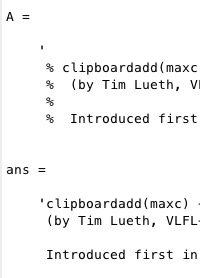% clipboardrem(maxc) - removes comment characters from the clipboard% (by Tim Lueth, FileMaker, 2017-JUL-07 as class: AUXILIARY PROCEDURES)% % Introduced first in SolidGeometry 4.0% % See also: clipboardadd% % C=clipboardrem([maxc])% === INPUT PARAMETERS ===% maxc: % === OUTPUT RESULTS ======% C: % % See also: clipboardadd%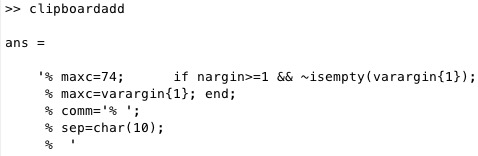% clipboardadd(maxc) - adds comment characters from the clipboard% (by Tim Lueth, VLFL-Lib, 2017-JUL-07 as class: AUXILIARY PROCEDURES)% % Introduced first in SolidGeometry 4.0% % See also: clipboardrem% % C=clipboardadd([maxc])% === INPUT PARAMETERS ===% maxc: % === OUTPUT RESULTS ======% C: % % See also: clipboardrem%
###### surfacesofSG(TR,alpha);- returns all OPEN surfaces that have a limited angle between their normal vectors% surfacesofSG(TR,alpha); - returns all OPEN surfaces that have a limited angle between their normal vectors% (by Tim Lueth, VLFL-Lib, 2017-JUL-06 as class: SURFACES)% % This fnctn can take several seconds to classify all OPEN surfaces of a % solid by using TR3neighborsAngle and neighborsAngleSurface.% When called without output parameter; drawing the surfaces take the % most time.% SGsurfaces returns CLOSED surfaces (Status of: 2017-07-07)% % Introduced first in SolidGeometry 4.0% % See also: neighborsAngleSurface, TR3neighborsAngle, SGsurfaces% % [FIL,k,FNL,NAL,NBL,Ai]=surfacesofSG(TR,[alpha]);% === INPUT PARAMETERS ===% TR: Solid Geometry or Triangulation% alpha: maximum angle difference between two facets; default is 1 ~ 60 % degree% === OUTPUT RESULTS ======% FIL: Index List for the Surface Indices% k: maximum value of FIL% FNL: Facet Normal List - TR3neighborsAngle% NAL: Neigbor Angle List - TR3neighborsAngle% NBL: Neigbor List - TR3neighborsAngle% Ai: Area of the indexed surfaces 1:k% % EXAMPLE: % load JACO_robot.mat% surfacesofSG(JC3);% % See also: neighborsAngleSurface, TR3neighborsAngle, SGsurfaces%
###### LaserInit- Initializes the USB-Serial Port and Requests Data from the Leuze Scanner% LaserInit - Initializes the USB-Serial Port and Requests Data from the Leuze Scanner% (by Tim Lueth & Sebastian Heininger, ROBOT-Lib, 2017-JUL-06)% % This is just an example how to use the Leuze Laser Scanner at the mac % computer. (Status of: 2017-07-06)% % Introduced first in SolidGeometry 4.0% % See also: USBhelp% % LaserInit% % See also: USBhelp%
###### SGinsideSurface(SG,fnum,wlim,ilim)- Checks the distance between the centers of the facets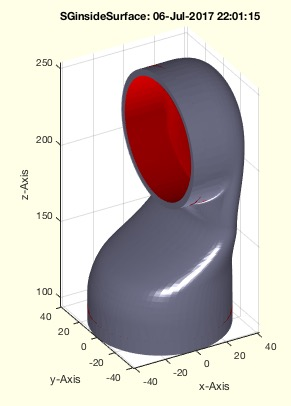% SGinsideSurface(SG,fnum,wlim,ilim) - Checks the distance between the centers of the facets% (by Tim Lueth, VLFL-Lib, 2017-JUL-06 as class: EXPERIMENTS)% % The fnctns SGcheckfacedist and SGinsidesurface are very similar. Both % ray trace the normal vector of a facet and detect self-penetration of % other facets. While SGcheckfacedist looks backwards, SGinsideSurface % looks forward along the normal vector. The only problem is the extreme % time-consuming quadratic growing effort. % In SGcheckfacedist there is almost never a need to process the original % data. But for SGinsidesurface there is wish to process the original % data. There we use a heuristics to accelerate the algorithm. First we % analyze freeeform surfaces using surfacesofSG, the we analyze just for % each of those surfaces ONE surface to have an opponent. (Status of: % 2017-07-07)% % Introduced first in SolidGeometry 4.0% % See also: SGcheckfacedist, SGinsidesurface, surfacesofSG% % [FIL,insFS]=SGinsideSurface(SG,[fnum,wlim,ilim])% === INPUT PARAMETERS ===% SG: Solid Geometry% fnum: reduce to face numbers; default is 3000;% wlim: warning limit; default is 0.7 mm% ilim: intended limit; distance that is considered as intended; default % is 0.1mm% === OUTPUT RESULTS ======% FIL: Facet surface index list% insFS: inside index of feature surface% % EXAMPLE: % load JACO_robot.mat% SG=SGsurfaces(JC3,3)% SGinsideSurface(SG)% % See also: SGcheckfacedist, SGinsidesurface, surfacesofSG%
###### diffangle(NL1,NL2,normfirst)- calculates the angle between to normal vector lists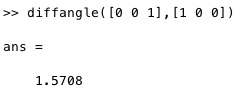% diffangle(NL1,NL2,normfirst) - calculates the angle between to normal vector lists% (by Tim Lueth, VLFL-Lib, 2017-JUL-06 as class: ANALYTICAL GEOMETRY)% % optimal for list processing. if normalization is required, the time is % about doubled (Status of: 2017-07-06)% % Introduced first in SolidGeometry 4.0% % See also: TR3neighborsAngle% % AL=diffangle(NL1,NL2,[normfirst])% === INPUT PARAMETERS ===% NL1: normal vector lists 1% NL2: normal vector lists 2% normfirst: if true; VLnorm is sued to norm the vectors first; % === OUTPUT RESULTS ======% AL: real(acos(dot(NL1(i,:),NL2(i,1))))% % EXAMPLE: NL1=rand(10,3); NL2=rand(10,3);% % % See also: TR3neighborsAngle%
###### SGcheckfacedist(SG,fnum,wlim,ilim)- Checks the distance between the centers of the facets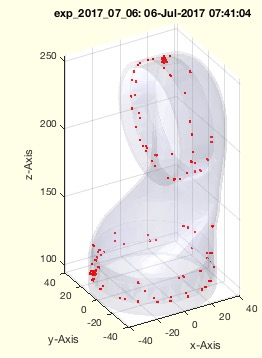% SGcheckfacedist(SG,fnum,wlim,ilim) - Checks the distance between the centers of the facets% (by Tim Lueth, VLFL-Lib, 2017-JUL-06 as class: EXPERIMENTS)% % Important test procedure for shrinked models and imported geometries. % Attention: Some geometries consist of intended overlapping structures % Attention: Some geometries consist of open surfaces (Status of: % 2017-07-06)% % Introduced first in SolidGeometry 4.0% % See also: exp_2017_07_06% % [ml,cpl]=SGcheckfacedist(SG,[fnum,wlim,ilim])% === INPUT PARAMETERS ===% SG: Solid Geometry% fnum: reduce to face numbers; default is 3000;% wlim: warning limit; default is 0.7 mm% ilim: intended limit; distance that is considered as intended; default % is 0.1mm% === OUTPUT RESULTS ======% ml: complete list of distances% cpl: complete list of cross points% % See also: exp_2017_07_06%
###### VLFLofSGT(SGN,N)- returns the VL and FL of the surface related to a fram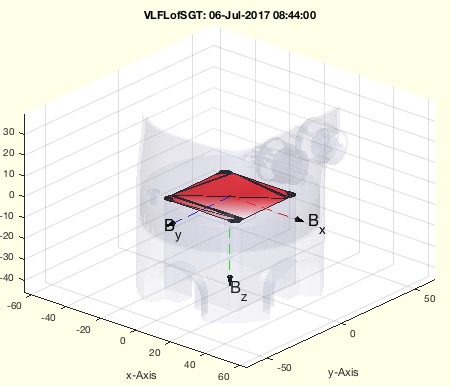% VLFLofSGT(SGN,N) - returns the VL and FL of the surface related to a fram% (by Tim Lueth, VLFL-Lib, 2017-JUL-06 as class: SURFACES)% % Introduced first in SolidGeometry 4.0% % See also: CVLofVLFL% % [VL,FL]=VLFLofSGT(SGN,N)% === INPUT PARAMETERS ===% SGN: Solid with Frames% N: Name of Frames% === OUTPUT RESULTS ======% VL: Vertex list; shortend% FL: FAcet list; shortend% % EXAMPLE: % loadweb JACO_robot.mat% VLFLofSGT(JC0,'B');% % See also: CVLofVLFL%
###### CVLofVLFL()- does the same as CVLfreeBoundaryVLFL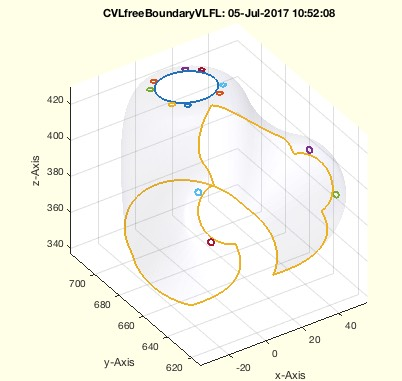% CVLofVLFL() - does the same as CVLfreeBoundaryVLFL% (by Tim Lueth, VLFL-Lib, 2017-JUL-06 as class: SURFACES)% % =======================================================================% OBSOLETE (2017-07-06) - USE FAMILY 'CVLfreeBoundaryVLFL' INSTEAD% =======================================================================% % Introduced first in SolidGeometry 4.0% % See also: [ CVLfreeBoundaryVLFL ] ; VLFLofSGT% % [CVL,TR]=CVLofVLFL([])% === OUTPUT RESULTS ======% CVL: % TR: % % See also: [ CVLfreeBoundaryVLFL ] ; VLFLofSGT%
###### CVLofSGT(SGN,N)- returns the CVL of the surface that belongs to a Frame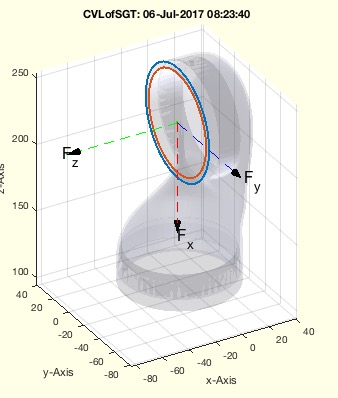% CVLofSGT(SGN,N) - returns the CVL of the surface that belongs to a Frame% (by Tim Lueth, VLFL-Lib, 2017-JUL-06 as class: SURFACES)% % Introduced first in SolidGeometry 4.0% % See also: VLFLofSGT% % CVL=CVLofSGT(SGN,N)% === INPUT PARAMETERS ===% SGN: Solid Geometry with Frames% N: Frame name% === OUTPUT RESULTS ======% CVL: Convtour Vertex List% % EXAMPLE: loadweb JACO_robot.mat% CVLofSGT(JC1,'F');% % See also: VLFLofSGT%
###### SGofsmbFullModelSimulation(SGi,Ti,tm,t)- returns a solid geometry for an arbitrary time value of a simulation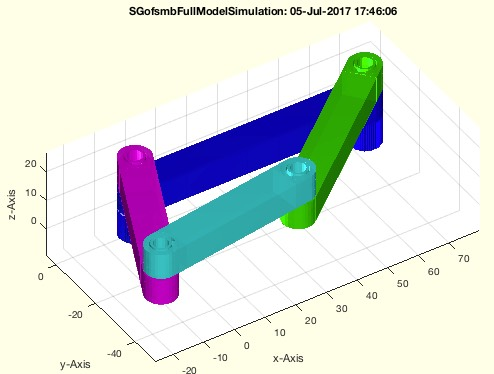% SGofsmbFullModelSimulation(SGi,Ti,tm,t) - returns a solid geometry for an arbitrary time value of a simulation% (by Tim Lueth, VLFL-Lib, 2017-JUL-05 as class: SIMMECHANICS INTERFACE)% % This fnctn is an add on to smbFullModelSimulation (Status of: % 2017-07-05)% % Introduced first in SolidGeometry 4.0% % See also: smbFullModelSimulation% % [SG,t]=SGofsmbFullModelSimulation(SGi,Ti,tm,t)% === INPUT PARAMETERS ===% SGi: List of Solid Geometries, result of smbFullModelSimulation% Ti: List of HT Frames, result of smbFullModelSimulation% tm: List of time points result of smbFullModelSimulation% t: desired time% === OUTPUT RESULTS ======% SG: Solid Geoemtry% t: Exact time of simulation% % EXAMPLE: % [~,BNi,SGi,Ti,tm]=smbFullModelSimulation(1);% SGofsmbFullModelSimulation(SGi,Ti,tm,0);% % See also: smbFullModelSimulation%
###### SGgrabcad- Simply opens the WWW-page of grabcad.com in the system browser% SGgrabcad - Simply opens the WWW-page of grabcad.com in the system browser% (by Tim Lueth, VLFL-Lib, 2017-JUL-05 as class: AUXILIARY PROCEDURES)% % Introduced first in SolidGeometry 4.0% % See also: SG_DBconnectors.mat, SG_Patientknee.mat% % SGgrabcad% % See also: SG_DBconnectors.mat, SG_Patientknee.mat%
###### CVLfreeBoundaryVLFL(VL,FL)- returns the CVL of the free boundary% CVLfreeBoundaryVLFL(VL,FL) - returns the CVL of the free boundary% (by Tim Lueth, VLFL-Lib, 2017-JUL-05 as class: SURFACES)% % Introduced first in SolidGeometry 4.0% % See also: freeBoundary, neighborsAngleSurface% % [CVL,TR2]=CVLfreeBoundaryVLFL(VL,FL)% === INPUT PARAMETERS ===% VL: Vertex List, or Solid Geometry, or triangulation% FL: Facet list, if VL is Vertex list% === OUTPUT RESULTS ======% CVL: Closed vertex contour list% TR2: triangulation% % EXAMPLE: % loadweb JACO_robot.mat% SG=JC61; [NAL,NBL]=TR3neighborsAngle(SG); % fi=neighborsAngleSurface(NAL,NBL,1,22000);% CVLfreeBoundary(SG.VL,SG.FL(fi,:))% % See also: freeBoundary, neighborsAngleSurface%
###### ELunique(ELo)- returns a unique list of all edges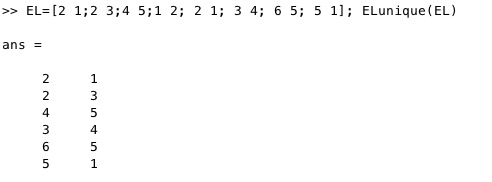% ELunique(ELo) - returns a unique list of all edges% (by Tim Lueth, VLFL-Lib, 2017-JUL-05 as class: EDGE LISTS)% % Makes sure that no edge is used twice!% More or less the same as ELuniqueofFL but will not change the ordner % and in case of doubles the direction of the first used edges is not % changed. (Status of: 2017-07-05)% % Introduced first in SolidGeometry 4.0% % See also: ELuniqueofFL% % EL=ELunique(ELo)% === INPUT PARAMETERS ===% ELo: Edge List or Facet List% === OUTPUT RESULTS ======% EL: Edge list sorted by first vertex index% % EXAMPLE: % EL=[2 1;2 3;4 5;1 2; 2 1; 3 4; 6 5; 5 1]; ELunique(EL)% % See also: ELuniqueofFL%
###### graphofEL(EL,n)- creates an undirected graph model from an edge list% graphofEL(EL,n) - creates an undirected graph model from an edge list% (by Tim Lueth, VLFL-Lib, 2017-JUL-05 as class: EDGE LISTS)% % This is the first fnctn to analyze surface models using graph theory.% It is extremely helpful to analyze the structures by this abstraction % level.% MATLAB supports since 2015b % undirected "graphs" and% directed "digraphs" (Status of: 2017-07-05)% % Introduced first in SolidGeometry 4.0% % See also: featureEdges% % G=graphofEL(EL,[n])% === INPUT PARAMETERS ===% EL: Edge list; must have unique entries!% n: optional number of vertices% === OUTPUT RESULTS ======% G: MATLAB's Graph struct using Edges and Nodes% % EXAMPLE: % EL=featureEdges(TR3ofSG(SGsample(25)),1)% graphofEL(EL)% graphofEL(featureEdges(TR3ofSG(SGsample(3)),1))% % See also: featureEdges%
###### neighborsAngleSurface(NAL,NBL,alpha,fi)- returns connected facets with respect to a feature angle% neighborsAngleSurface(NAL,NBL,alpha,fi) - returns connected facets with respect to a feature angle% (by Tim Lueth, VLFL-Lib, 2017-JUL-05 as class: SURFACES)% % The required parameter NAL,NBL are calculated using TR3neighborsAngle. % Since TR3neighborsAngle can be slow, the fnctns are separated to % accelerate the repeatet use of neighborsAngleSurface.% % (Status of: 2017-07-05)% % Introduced first in SolidGeometry 4.0% % See also: TR3neighborsAngle% % [fa,FCL]=neighborsAngleSurface(NAL,NBL,alpha,fi)% === INPUT PARAMETERS ===% NAL: =TR3neighborsAngle(TR3)% NBL: =neighbors(TR3), also delivered by [NAL,NBL]=TR3neighborsAngle(TR3)% alpha: feature angle% fi: starting facet index (can be several)% === OUTPUT RESULTS ======% fa: facet index with respect to NAL,NBL of (TR3)% FCL: Facet connection list; optional% % EXAMPLE: % SG=SGsample(25);% [NAL,NBL]=TR3neighborsAngle(SG); fi=neighborsAngleSurface(NAL,NBL,1,22);% VLFLplot(SG.VL,SG.FL(fi,:))% % See also: TR3neighborsAngle%
###### TR3featureEdges(TR3,alpha)- returns feature edge List plus edge attached facets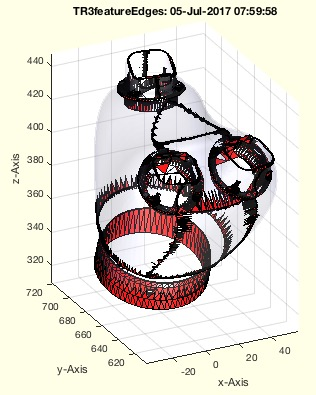% TR3featureEdges(TR3,alpha) - returns feature edge List plus edge attached facets% (by Tim Lueth, VLFL-Lib, 2017-JUL-05 as class: SURFACES)% % Auxiliary fnctn as starting point: % Simply calls featureEdges. It is not using edgeAttachments but an own % solution% (Status of: 2017-07-05)% % Introduced first in SolidGeometry 4.0% % [EL,Fi]=TR3featureEdges(TR3,alpha)% === INPUT PARAMETERS ===% TR3: Triangulation% alpha: feature edge angle% === OUTPUT RESULTS ======% EL: Feature Edge List% Fi: Index of TR3.ConnectivityList% % EXAMPLE: % TR3featureEdges(SGsample(25),1)%
###### TR3neighborsAngle(TR3)- returns the angle difference between a facet an its neigbors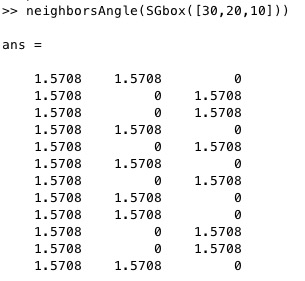% TR3neighborsAngle(TR3) - returns the angle difference between a facet an its neigbors% (by Tim Lueth, Video-Lib, 2017-JUL-04 as class: SURFACES)% % Calculated the difference angle between a surface and its neighbor % surfaces.% Typically used in combination with/to create the input for % fnctn neighborsAngleSurface (Status of: 2017-07-05)% % Introduced first in SolidGeometry 4.0% % See also: neighborsAngleSurface, diffangle% % [NAL,NBL,FNL]=TR3neighborsAngle(TR3)% === INPUT PARAMETERS ===% TR3: Triangulation or SG% === OUTPUT RESULTS ======% NAL: Facet Normal Angle List wrt to NBL% NBL: Neighbor List = neighbors(TR3)% FNL: Facet normal List = faceNormal(TR3)% % See also: neighborsAngleSurface, diffangle%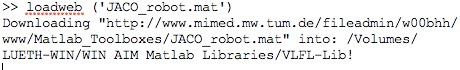% loadweb (mname,overwr,urlp) - loads mat files from an web url% (by Tim Lueth, VLFL-Lib, 2017-JUL-03 as class: AUXILIARY PROCEDURES)% % This fnctn reduces the size of the SG-Lib Toolbox since the '.mat' % files and the directories can be downloaded once into the user % directory.% if parameter overwr=false; the filename will be "downloaded_mname"% if parameter overwr=true; the filename will be "mname"% Try: % 'AArrufo_surf.mat' (3.6 MByte)% 'JACO_robot.mat' (6.1 MByte)% 'AIM_SGrobot.mat' (0.1 MByte)% 'AIM_Patientmodel.mat' ( 5MByte)% 'ModulKegelradwelle_1.mat' (0.2 MByte) (Status of: 2017-07-03)% % Introduced first in SolidGeometry 4.0% % See also: load, webread% % loadweb(mname,[overwr,urlp])% === INPUT PARAMETERS ===% mname: name and extension of the file% overwr: true=overwrite; default is false.% urlp: web path; default is % 'https://www.mec.ed.tum.de/fileadmin/w00cbp/mimed/Matlab_Toolboxes/'% % EXAMPLE: % loadweb('JACO_robot.mat') % loads the downloaded file% loadweb('JACO_robot.mat',true) % replaces the original file by the % downloaded file% % See also: load, webread%